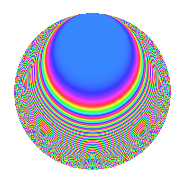# Properties

 Label 27.3.fLevel 27 Weight 3 Character orbit f Rep. character $$\chi_{27}(2,\cdot)$$ Character field $$\Q(\zeta_{18})$$ Dimension 30 Newforms 1 Sturm bound 9 Trace bound 0

# Related objects

## Defining parameters

 Level: $$N$$ = $$27 = 3^{3}$$ Weight: $$k$$ = $$3$$ Character orbit: $$[\chi]$$ = 27.f (of order $$18$$ and degree $$6$$) Character conductor: $$\operatorname{cond}(\chi)$$ = $$27$$ Character field: $$\Q(\zeta_{18})$$ Newforms: $$1$$ Sturm bound: $$9$$ Trace bound: $$0$$

## Dimensions

The following table gives the dimensions of various subspaces of $$M_{3}(27, [\chi])$$.

Total New Old
Modular forms 42 42 0
Cusp forms 30 30 0
Eisenstein series 12 12 0

## Trace form

 $$30q$$ $$\mathstrut -\mathstrut 6q^{2}$$ $$\mathstrut -\mathstrut 6q^{3}$$ $$\mathstrut -\mathstrut 6q^{4}$$ $$\mathstrut -\mathstrut 15q^{5}$$ $$\mathstrut -\mathstrut 18q^{6}$$ $$\mathstrut -\mathstrut 6q^{7}$$ $$\mathstrut -\mathstrut 9q^{8}$$ $$\mathstrut +\mathstrut O(q^{10})$$ $$30q$$ $$\mathstrut -\mathstrut 6q^{2}$$ $$\mathstrut -\mathstrut 6q^{3}$$ $$\mathstrut -\mathstrut 6q^{4}$$ $$\mathstrut -\mathstrut 15q^{5}$$ $$\mathstrut -\mathstrut 18q^{6}$$ $$\mathstrut -\mathstrut 6q^{7}$$ $$\mathstrut -\mathstrut 9q^{8}$$ $$\mathstrut -\mathstrut 3q^{10}$$ $$\mathstrut -\mathstrut 6q^{11}$$ $$\mathstrut -\mathstrut 15q^{12}$$ $$\mathstrut -\mathstrut 6q^{13}$$ $$\mathstrut -\mathstrut 15q^{14}$$ $$\mathstrut -\mathstrut 9q^{15}$$ $$\mathstrut -\mathstrut 18q^{16}$$ $$\mathstrut -\mathstrut 9q^{17}$$ $$\mathstrut +\mathstrut 63q^{18}$$ $$\mathstrut -\mathstrut 3q^{19}$$ $$\mathstrut +\mathstrut 213q^{20}$$ $$\mathstrut +\mathstrut 132q^{21}$$ $$\mathstrut -\mathstrut 42q^{22}$$ $$\mathstrut +\mathstrut 120q^{23}$$ $$\mathstrut +\mathstrut 144q^{24}$$ $$\mathstrut -\mathstrut 15q^{25}$$ $$\mathstrut -\mathstrut 90q^{27}$$ $$\mathstrut -\mathstrut 12q^{28}$$ $$\mathstrut -\mathstrut 168q^{29}$$ $$\mathstrut -\mathstrut 243q^{30}$$ $$\mathstrut +\mathstrut 39q^{31}$$ $$\mathstrut -\mathstrut 360q^{32}$$ $$\mathstrut -\mathstrut 207q^{33}$$ $$\mathstrut +\mathstrut 54q^{34}$$ $$\mathstrut -\mathstrut 252q^{35}$$ $$\mathstrut -\mathstrut 360q^{36}$$ $$\mathstrut -\mathstrut 3q^{37}$$ $$\mathstrut -\mathstrut 84q^{38}$$ $$\mathstrut +\mathstrut 15q^{39}$$ $$\mathstrut -\mathstrut 33q^{40}$$ $$\mathstrut +\mathstrut 228q^{41}$$ $$\mathstrut +\mathstrut 486q^{42}$$ $$\mathstrut -\mathstrut 96q^{43}$$ $$\mathstrut +\mathstrut 639q^{44}$$ $$\mathstrut +\mathstrut 477q^{45}$$ $$\mathstrut -\mathstrut 3q^{46}$$ $$\mathstrut +\mathstrut 399q^{47}$$ $$\mathstrut +\mathstrut 453q^{48}$$ $$\mathstrut -\mathstrut 78q^{49}$$ $$\mathstrut +\mathstrut 303q^{50}$$ $$\mathstrut +\mathstrut 36q^{51}$$ $$\mathstrut -\mathstrut 9q^{52}$$ $$\mathstrut -\mathstrut 54q^{54}$$ $$\mathstrut -\mathstrut 12q^{55}$$ $$\mathstrut -\mathstrut 393q^{56}$$ $$\mathstrut -\mathstrut 192q^{57}$$ $$\mathstrut +\mathstrut 129q^{58}$$ $$\mathstrut -\mathstrut 474q^{59}$$ $$\mathstrut -\mathstrut 846q^{60}$$ $$\mathstrut +\mathstrut 138q^{61}$$ $$\mathstrut -\mathstrut 900q^{62}$$ $$\mathstrut -\mathstrut 585q^{63}$$ $$\mathstrut -\mathstrut 51q^{64}$$ $$\mathstrut -\mathstrut 411q^{65}$$ $$\mathstrut -\mathstrut 423q^{66}$$ $$\mathstrut +\mathstrut 354q^{67}$$ $$\mathstrut +\mathstrut 99q^{68}$$ $$\mathstrut +\mathstrut 99q^{69}$$ $$\mathstrut +\mathstrut 489q^{70}$$ $$\mathstrut +\mathstrut 315q^{71}$$ $$\mathstrut +\mathstrut 720q^{72}$$ $$\mathstrut -\mathstrut 66q^{73}$$ $$\mathstrut +\mathstrut 321q^{74}$$ $$\mathstrut +\mathstrut 255q^{75}$$ $$\mathstrut +\mathstrut 258q^{76}$$ $$\mathstrut +\mathstrut 201q^{77}$$ $$\mathstrut +\mathstrut 180q^{78}$$ $$\mathstrut +\mathstrut 30q^{79}$$ $$\mathstrut +\mathstrut 36q^{81}$$ $$\mathstrut -\mathstrut 12q^{82}$$ $$\mathstrut -\mathstrut 33q^{83}$$ $$\mathstrut -\mathstrut 588q^{84}$$ $$\mathstrut -\mathstrut 261q^{85}$$ $$\mathstrut -\mathstrut 258q^{86}$$ $$\mathstrut -\mathstrut 279q^{87}$$ $$\mathstrut -\mathstrut 642q^{88}$$ $$\mathstrut +\mathstrut 72q^{89}$$ $$\mathstrut +\mathstrut 288q^{90}$$ $$\mathstrut +\mathstrut 96q^{91}$$ $$\mathstrut -\mathstrut 3q^{92}$$ $$\mathstrut +\mathstrut 591q^{93}$$ $$\mathstrut -\mathstrut 861q^{94}$$ $$\mathstrut +\mathstrut 681q^{95}$$ $$\mathstrut +\mathstrut 270q^{96}$$ $$\mathstrut -\mathstrut 582q^{97}$$ $$\mathstrut +\mathstrut 882q^{98}$$ $$\mathstrut +\mathstrut 513q^{99}$$ $$\mathstrut +\mathstrut O(q^{100})$$

## Decomposition of $$S_{3}^{\mathrm{new}}(27, [\chi])$$ into irreducible Hecke orbits

Label Dim. $$A$$ Field CM Traces $q$-expansion
$$a_2$$ $$a_3$$ $$a_5$$ $$a_7$$
27.3.f.a $$30$$ $$0.736$$ None $$-6$$ $$-6$$ $$-15$$ $$-6$$